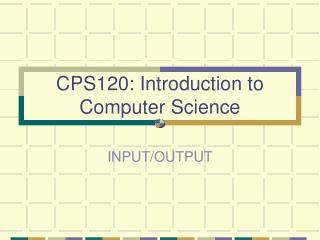DownloadDownload PresentationCPS120: Introduction to Computer Science

# CPS120: Introduction to Computer Science

Télécharger la présentation## CPS120: Introduction to Computer Science

- - - - - - - - - - - - - - - - - - - - - - - - - - - E N D - - - - - - - - - - - - - - - - - - - - - - - - - - -
##### Presentation Transcript

1. CPS120: Introduction to Computer Science INPUT/OUTPUT

2. Input/Output Structures • In our pseudocode algorithms we have used the expressions Read and Write • High-level languages view input data as a stream of characters divided into lines

3. Console I/O • console I/O refers to using the keyboard and screen as the standard input and output devices, respectively.

4. C-In (cin) • cin is a stream of data flowing from an input device such as the keyboard (considered to be the standard input device) into your program.

5. Input Operations • The operator >> is known as the input operator. It is also known as the extraction operator • You use the input operator in statements like, cin >> numItems; which would allow the user to input a value to be stored in the variable numItems.

6. Inputting Words • >> can be used -- but once it hits a space or a tab, the rest of the string is ignored • Use the get function for strings that contain ‘white space’

7. Complexities of Word Input • Some things are done automatically with >> • get does not skip over line breaks and spaces • If the user enters a string longer than the length specified in the call to the get function, the remaining characters are left in the input stream • get always ignores the new line character (‘\n’) and leaves it in the stream • Use the ignore function to flush the contents of the input stream cin.ignore(80, ‘\n’);

8. Output Operations • The operator << is known as the output operator. It is also known as the insertion operator • You use the output operator in statements like:cout << "Hello world";

9. C-Out (cout) • cout is a stream which represents data flowing from one place to another. • The statement: cout << "Hello world"; causes data to flow from your program to the screen. • The stream, cout, leads to what your computer considers to be its standard output device (usually the screen)

10. Line Spacing • In order to end a line in an output statement you may use the new line character, \n, instead of endl. • Examples: cout << "Hello world" << '\n'; cout << "Hello world" << "\n"; cout << "Hello world\n"; • These are practically equivalent to: cout << "Hello world" << endl;

11. Escape Sequences • Other useful "escape sequences" (since the \ is the escape operator) are: \t to generate a tab \\ to print a backslash \' to print a single quote \" to print a double quote

12. Using setf and unsetf • Each stream has format options that can be changed OPTION DESCRIPTION left Left-justifies the output right Right-justifies the output showpoint Displays decimal point and trailing zeros forfloats uppercase Displays e in scientific as E showpos Displays a leading plus sign scientific Displays floating point number scientifically fixed Displays floating-point in normal notation

13. Using Format Options • Format options are set immediately prior to the COUT statement float x = 24.0; cout << x << ‘\n’; // displays 24 cout.setf(ios::showpoint); cout << x << ‘\n’; // displays 24.00000 cout.unsetf(ios::showpoint); cout << x << ‘\n’; // displays 24

14. Using Manipulators • You must include the <iomanip.h> header file at the top of your program in order to use the setprecision, setw, and other manipulators. You must use place the following compiler directive at the top of your program.#include <iomanip.h> • I/O manipulators are placed directly in the output statement cout << setprecision(2) << price << ‘\n’;

15. Setting Precision • The setprecision manipulator allows you to limit the number of digits that are displayed when a numeric data type is displayed: cout << setprecision(2) << price << '\n'; only allows the leading two digits of the value stored in the variable, price, to be displayed

16. More Precisely • If the fixed format was set previously with the statement: cout.setf(ios::fixed); then the setprecision(2) manipulator would have the effect of rounding or truncating price (and all future floating-point values in the cout stream) to the hundredths place

17. Field Width • The setw manipulator controls the width of the field when displaying a value. The statement: cout << setw(10) << umEndow << endl; sets the width of the field allocated for the variable, umEndow, to 10 characters

18. Formatted Output -- Currency cout.setf(ios : : fixed) • Print “fixed point” form, not in exponential form cout.setf(ios : : showpoint) • Says to always print the decimal point cout.precison(2) • Says to print out the two most significant decimal digits, after rounding to this precision